×

Search anything:Circular Linked list is a complex form of a linked list data structure as within a circular linked list the last node points to the first node of the list.

In other words, the null pointer of the last node is replaced with the address of its first node.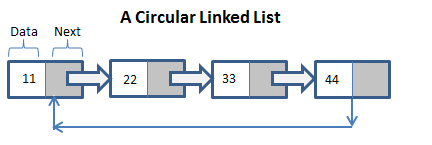A circular linked list was proposed due to the several advantages it contained over a linked list, such as:

1. For any node in a circular list, it became possible to reach another node in the circular list
2. If we traverse through the circular list, then we would eventually reach the starting node.
3. Deletion of a node became easy and convenient.
4. Operations such as splitting and concatenation became more efficient.
5. The circular linked list can also be implemented to create other data structures such as the Fibonacci Heap.

A circular linked does not have a beginning node or an ending node , by common convention we create the beginning and the ending node . By another common convention is to let the external pointer of the last node to point to the first node- the next of the last is the first.

There is a disadvantage to the circular linked list. The disadvantage can occur if there is no proper care in processing i.e. the circular list can get into an infinite loop. In the processing stage of a circular linked list , it is important that we are able to identify the end of the list by placing a node called the list head. This results in the list never being empty.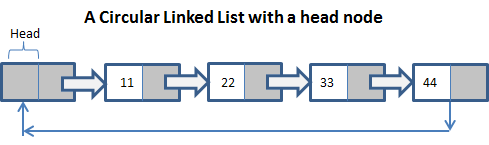## Operations:

Operations that can be performed with the help of a circular linked list are:

1. Insertion
2. Deletion
3. Traversal and Displaying

Before we perform the operations we need to Create a ‘Node’ class which helps us instantiate the circular list.
The implementation of the node class can be done in the following steps:

1. Define the ‘node’ class consisting of two members such as ‘data’ and ‘next’.
2. ‘data’ must be of the data-type Integer.
3. The ‘next’ member must be of the data-type ‘Node’.

Code(Java):

``````static class Node
{
int data;
Node next;
};
``````

## Insertion:

Insertion of a node in the circular list can be performed in three ways :

1. Insertion of the node at the beginning of the list.
2. Insertion of the node at the end of the list.
3. Insertion of the node at a specified position of the list.

### Insertion of the node at the beginning of the list :

This operation can be performed in the following steps

1. First step is to create a newNode with some data.
2. Then, checking whether the list is empty or not (last==null).
3. If the list is Empty, then call a function ‘IfEmpty’, which basically adds the newNode to the beginning of the list.
4. If the list is Not-Empty then define a temporary variable called ‘temp’ and initialize it to head.
5. The next step is to keep assigning the 'temp' to it's next node until the last node is reached.
6. The last step is to set ‘the next of newNode to head’,’head to newNode’ ,and ‘the next of temp to head’.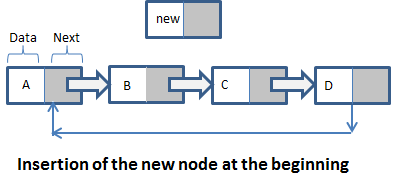After Insertion of the new Node: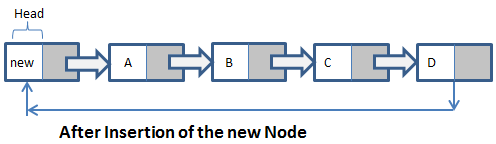Code(java):
``````static class Node
{
int data;
Node next;
};
static Node IfEmpty(Node last, int data)
{
if (last != null)
return last;

Node temp = new Node();

temp.data = data;
last = temp;

last.next = last;
return last;
}
static Node AtBeginning(Node last, int data)
{
if (last == null)
return IfEmpty(last, data);

Node temp = new Node();

temp.data = data;
temp.next = last.next;
last.next = temp;

return last;
}
``````

### Insertion of the node at the end of the list:

This operation can be performed in the following steps:

1. First step is to create a newNode with some data.
2. Then, checking whether the list is empty or not (last==null).
3. If the list is Empty, then call a function ‘IfEmpty’, which basically adds the newNode to the end of the list.
4. If the list is Not-Empty then define a temporary variable called ‘temp’ and initialize it to head.
5. The next step is to keep assigning the 'temp' to it's next node until the last node is reached.
6. The last step would be to set ‘the next of the temp to newNode’ and ‘next of newNode to head’.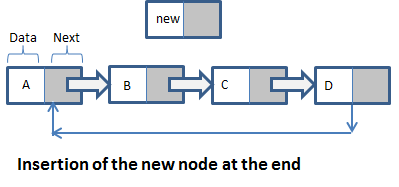After Inserting the node at the end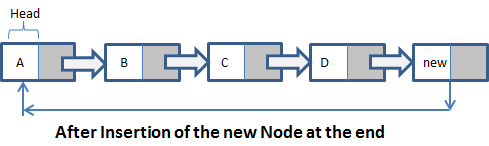``````static class Node
{
int data;
Node next;
};
static Node IfEmpty(Node last, int data)
{
if (last != null)
return last;

Node temp = new Node();

temp.data = data;
last = temp;

last.next = last;
return last;
}
static Node AtEnd(Node last, int data)
{
if (last == null)
return IfEmpty(last, data);

Node temp = new Node();

temp.data = data;
temp.next = last.next;
last.next = temp;
last = temp;

return last;
}
``````

### Insertion of the node at a specified position of the list:

This operation can be performed in the following steps:

1. First step is to create a newNode with some data.
2. Then, checking whether the list is empty or not (last==null).
3. If the list is Empty, then call a function ‘IfEmpty’, which basically adds the newNode to the end of the list.
4. If the list is Not-Empty then define a temporary variable called ‘temp’ and initialize it to head.
5. The next step is to keep assigning the 'temp' to it's next node until it has reached the node after which we want to insert the new node.
6. The following step is to check whether the new Node has reached the last node or not, if it has reached the last then the node is not found in the list and eventually we end the program.
7. If the program is not terminated, then move the ‘temp’ to the next node.
8. If the ‘temp’ reaches the desired node , then we need to check whether it is the last node.
9. If the ‘temp’ is the last node, then we set ‘the next of temp to newNode’ and ‘the next of newNode to head’
10. If the ‘temp’ is not the last node, then we set ‘the next of newNode to the next of temp’ and ‘the next of temp to newNode’.

The Following images illustrates the steps: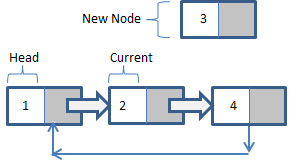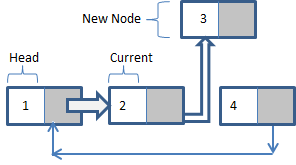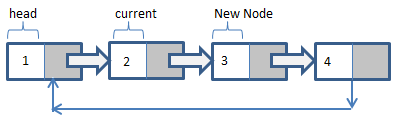Code(Java):

``````static class Node
{
int data;
Node next;
};
static Node AddMiddle(Node last, int data, int item)
{
if (last == null)
return null;

Node temp, k;
k = last.next;
do
{
if (k.data == item)
{
temp = new Node();
temp.data = data;
temp.next = k.next;
k.next = temp;

if (k == last)
last = temp;
return last;
}
k = k.next;
} while(k != last.next);

System.out.println(item + " not present in the list.");
return last;
}
``````

## Deletion:

deletion of a node in the circular list can be performed in three ways :

1. Deletion of the node at the beginning of the list.
2. Deletion of the node at the end of the list.
3. Deletion of the node at a specified position of the list.

### Deletion of the node at the beginning of the list:

This operation can be performed in the following steps:

1. The first step is to check whether the list is Empty or not (Head==NULL).
2. If Empty then deletion is not possible.
3. If the list is not Empty, then initialize two nodes called ‘temp1’ and ‘temp2’ and assign them the value of head.
4. The next step is to check if the list is having only one node.
5. If True then set the value of head to NULL and delete temp1.
6. If False then move temp1 till the last node.
7. Then set ‘the value of head to the next of temp2’ , ‘ the next of temp1 to head’ and delete temp2.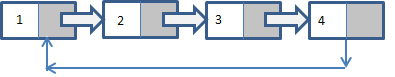After the deletion of the starting node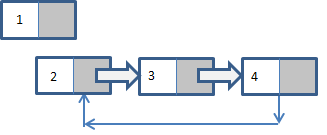Code(Java):

``````static class Node
{
int data;
Node next;
};
public Node tail = null;
public void deleteStart() {

return;
}
else {
}
else {
}
}
}
``````

### Deletion of the node at the end of the list:

This operation can be performed in the following steps:

1. The first step is to check whether the list is Empty or not (Head==NULL).
2. If Empty then deletion is not possible.
3. If the list is not Empty, then initialize two nodes called ‘temp1’ and ‘temp2’ and assign temp1 with the value of head.
4. The next step is to check if the list is having only one node.
5. If True , then set ‘the value of head to null’ and delete temp1, and then finish executing the program.
6. If False, then set ‘the value of temp2 to temp1’ and iterate temp1 to it’s next node. Repeat the process till temp1 has reached the last node.
7. The final step would be to set ‘the next of temp2 to head’ and delete temp1.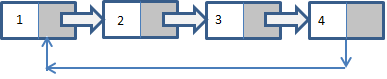After the deletion of the node from the end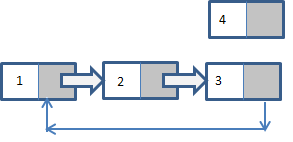Code(java):

``````static class Node
{
int data;
Node next;
};
{

{
System.out.printf("\nList is empty");
return null;
}

if (current.next == current)
{
return null;
}
{
previous = current;
current = current.next;
}

previous.next = current.next;

}
``````

### Deletion of the node at a specified position of the list:

This operation can be performed in the following steps:

1. The first step is to check whether the list is Empty or not (Head==NULL).
2. If Empty then deletion is not possible.
3. If the list is not Empty, then initialize two nodes called ‘temp1’ and ‘temp2’ and assign temp1 with the value of head.
4. The next step is to keep iterating the value of temp1 until it reaches the exact node to be deleted or till the last node.
5. Before moving the value of temp1 it is essential to set ‘the value of temp2 to temp1’.
6. If temp1 has reached the last node then Deletion is not possible.
7. If it has reached the node we want to delete then check whether it is the only node.
8. If the list has only one node and that is the node to be deleted then we need to set ‘the value of head to null’ and delete the node temp1.
9. If the circular list has multiple nodes then we need to check if the node ‘temp1’ is the first node or not.
10. If ‘temp1’ is the 1st node then set ‘the value of temp2 to head’ and keep iterating ‘temp2’ till the last node .
11. Then set ‘the value of head to the next of head’ , ‘ the next of temp2 to the value of head’ and then delete temp1.
12. If ‘temp1’ is not the first node then check whether it is the last node.
13. If ‘temp1’ is the last node then set ‘the next of temp2 to head’ and delete ‘temp1’.
14. If ‘temp1’ is not the first or last node then set ‘the next of temp2 to the next of temp1’ and delete the value of temp1.

The following illustrates the operations above: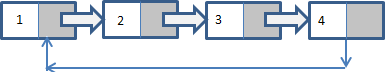After the insertion of the node at a specified position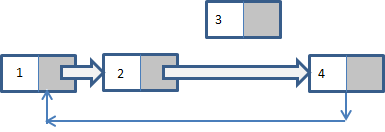Code(Java)

``````static class Node
{
int data;
Node next;
};
{
int count = 0;
{
return 0;
}
else
{
do
{
current = current.next;
count++;
}
return count;
}
static Node DeleteAtPosition( Node head, int index)
{
int count = 1;
{
System.out.printf("list is empty\n");
return null;
}

if (index >= len || index < 0)
{
return null;
}
if (index == 0)
{
}
while (len > 0)
{
if (index == count)
{
previous.next = next.next;
}
previous = previous.next;
next = previous.next;
len--;
count++;
}
}
{
{
System.out.printf("\nEmpty List");
return null;
}
if (previous.next == previous)
{
return null;
}
{
previous = previous.next;
next = previous.next;
}
previous.next = next.next;

}
``````

## Traversal and Displaying:

Traversing a circular linked list can be done in the following steps:

1. The first step is to check whether the list is Empty or not (Head==NULL).
2. If the list is Empty then finish executing the program.
3. If the list is not Empty then define a node pointer called ‘temp’ and initialize it with the value of the head node.
4. Keep iterating through the list and printing the value of the nodes by setting ‘the value of temp to the value of the data of the nodes’ until ‘temp’ has reached the last node.
5. Finally print the values by setting ‘the value of temp to the value data’.

Code(Java);

``````static class Node
{
int data;
Node next;
};
static void traverse(Node last)
{
Node p;
if (last == null) {
System.out.println("Circular list is empty.");
return;
}
p = last.next;
do{
System.out.print(p.data + "==>");
p = p.next;
}
while(p != last.next);
if(p == last.next)
System.out.print(p.data);
System.out.print("\n\n");
}
``````

## Time Complexity For All Operations:

Particulars Time Complexity Space Complexity
creation O(1) O(1)
Insertion O(n) O(1)
Traversing O(n) O(1)
Searching O(n) O(1)
Deletion of a node O(n) O(1)
Deletion of the Linked list O(1) O(1)

## Applications:

1. The cicular linked list is the main idea behind the working of the Application shifting which is present in the OS. All the applications are present in an loop with the help of the circular linked list.
2. They can also be implemented in many multiplayer games as in they manipulate the order in which the chance is provieded to the user.
3. They form the foundation of other data Structures such as Circular Queue.

## Questions:

Circular list

Circular list

#### In a Circular list , the modification of the nodes present in the list for insertion is a:

Two Pointer manipulation
One pointer manipulation
Three pointer manipulation
Static memory assignment